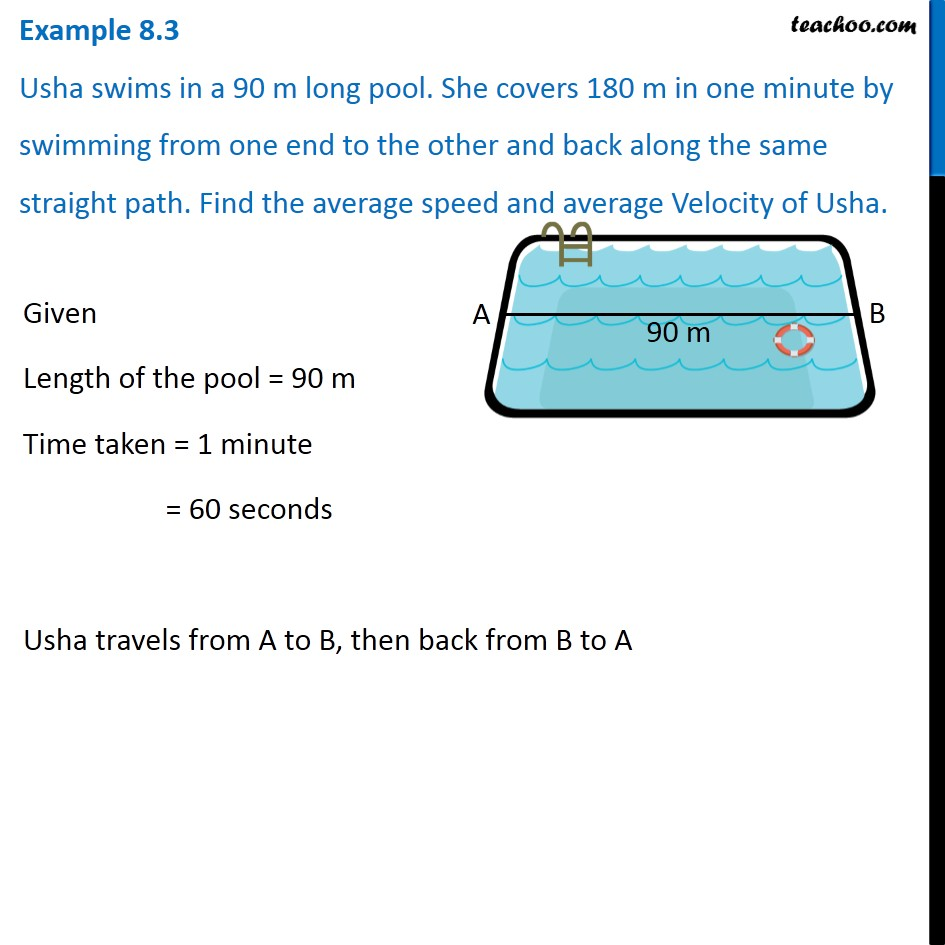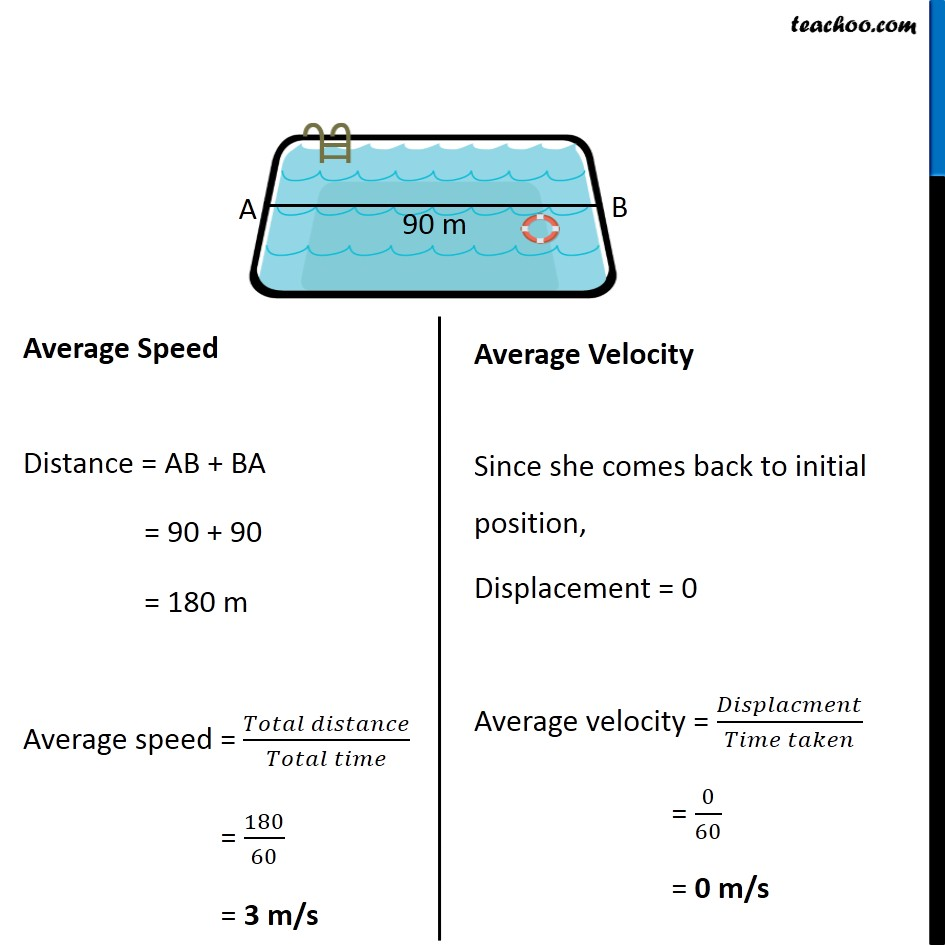Examples from NCERT Book

Class 9
Chapter 8 Class 9 - MotionGet live Maths 1-on-1 Classs - Class 6 to 12

### Transcript

Example 8.3 Usha swims in a 90 m long pool. She covers 180 m in one minute by swimming from one end to the other and back along the same straight path. Find the average speed and average Velocity of Usha. Given Length of the pool = 90 m Time taken = 1 minute = 60 seconds Usha travels from A to B, then back from B to A Distance travelled = AB + BA = 90 + 90 = 180 m Since she comes back to initial position, Displacement = 0 Average Speed Distance = AB + BA = 90 + 90 = 180 m Average speed = (𝑇𝑜𝑡𝑎𝑙 𝑑𝑖𝑠𝑡𝑎𝑛𝑐𝑒)/(𝑇𝑜𝑡𝑎𝑙 𝑡𝑖𝑚𝑒) = 180/60 = 3 m/s Average Velocity Since she comes back to initial position, Displacement = 0 Average velocity = 𝐷𝑖𝑠𝑝𝑙𝑎𝑐𝑚𝑒𝑛𝑡/(𝑇𝑖𝑚𝑒 𝑡𝑎𝑘𝑒𝑛) = 0/60 = 0 m/s